Study Guide

# Solving Circuit Questions

## Solving Circuit Questions

Using Ohm's Law, we can solve the world's simplest circuit.What in the name of Karl Friedrich Gauss' ghost, you may be asking, is that? Well, that's a circuit. It'd be a pain to draw batteries and loops of wire and light bulbs all the time, so physicists have come up with a graphical shorthand for any possible addition to a circuit. The ones we've learned about already are:We can trace the path of current by moving around the loop—the battery adds energy to charges, they move effortlessly through the wire until they hit the resistor, and then travel through the resistor, dissipating energy as heat as they do, before returning to the battery to start the whole cycle over again. Current flows from the positive end of the battery, through the loop, and into the negative end (in this case, clockwise):From Ohm's Law, we know the voltage drop across the resistor is IR, which is exactly equal to the potential added by the battery, V. The bottom of the resistor is at ground—the lowest potential point of the circuit, equivalent to our point of zero potential energy. Why is it at ground? Well, because the charge that passes through this resistor's voltage drop has zero potential difference with respect to the bottom of the battery.

The power dissipated in the resistor is given by:

P = IV = I2R

While a lot of more complicated circuits use little cylindrical resistors as a way to change the potential at a point in the loop, in a simple circuit like this one, the resistor, which can be something thing like an incandescent light bulb, are more likely represents the end goal. Then all that power being dissipated is turning electrical energy into heat and light. A 60 W bulb plugged into the 120 V wall socket of your bedroom will need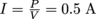of current.

But what if we want two light bulbs? There are two very different ways to attach a second resistor to our circuit:The first example is called a series connection, where one resistor leads to the next and current only has one possible path to flow through. The second example is a parallel connection, where each charge has to choose which branch and which resistor to travel through. Current will flow out of the battery, split and travel through the two branches, then reunite and re-enter the battery—it won't ever get trapped looping around from resistor 1 to resistor 2.

In both cases, we can apply Ohm's Law once we've reduced the circuit to its simplest form: one battery, one resistor. There are two ways to do this, depending on the way the second resistor is attached.

When in series, resistance is purely additive—all we have to do is add the two resistors' R values together to get one big equivalent super resistor.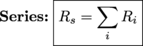In parallel, the splitting current means the net resistance actually goes down, as given by the inverse of a sum of the resistors' inverses.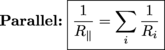Once we've simplified, we can use Ohm's Law to find the net voltage drop or total current. But if we want to know specific things about each circuit element instead of the overall picture, we need a couple more rules.

### Kirchhoff's Circuit Laws

There are two laws developed by Gustav Kirchhoff that are essential for solving circuit problems.

Kirchhoff's Current Law (KCL) states that the current that flows into any node (junction point) of a circuit is exactly equal to the current that flows out of that node. In a series connection, with no nodes, this means the current through both resistors must be exactly the same. In a parallel connection, the current leaving the battery must split and travel through both resistors before recombining.Kirchhoff's Voltage Law (KVL) states that the net voltage drop around any closed loop (viable current path) in a circuit must be zero. This is essentially conservation of energy—any energy added to the system by the battery must be removed by the resistors, and no more.

In a series connection, this means the voltage added by the battery is dissipated over both resistors, so that the sum of the voltage drop across R1 and the voltage drop across R2 is exactly the voltage added by the battery. In a parallel connection, the voltage drop across both resistors must be equal to each other and equal to the battery voltage, since both resistors offer an independent path for current to follow.TL;DR: In series, the current through the resistors is the same. In parallel, the voltage drop across the resistors is the same.

KCL and KVL can combine (Voltron style) to give us an equation that describes the behavior of each loop of the circuit. In series, we can use Ohm's Law and KVL to get:

V – IR1IR2 = 0

or

V – IRs = 0

In parallel, we can use Ohm's Law, KCL, and KVL to get:

V – I1R1 = 0

VI2R2 = 0

or

V – ItotR|| = 0

Let's see an example of the parallel case. If V = 5 V, R1 = 100Ω, and R2 = 200Ω, what is the voltage drop across each resistor? What is the current through each resistor? What is the current provided by the battery? What is your favorite color?The voltage drop is easy—each resistor provides a viable and mutually exclusive path for current, so the drop across both resistors must be equal to the voltage provided by the battery (5 V), as stated by KVL.

We can then use Ohm's Law to find the current in each resistor:Finally, we know the current provided by the battery must equal the sum of these two currents, as stated by KCL—Itot enters the parallel junction, and I1 and I2 leave. Therefore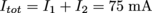Let's check this. If we replace the two parallel resistors with one equivalent resistor,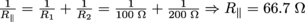. The current through R|| is equal to the current provided by the battery, and given by Ohm's Law: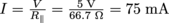.

You've just solved your first circuit. Move over, Tony Stark—there's a new engineer in town.

### Common Mistakes

Kirchhoff's Current Law applies to nodes; Kirchhoff's Voltage Law to loops. Remember what each says (current in equals current out / voltage applied equals voltage lost) and you'll cruise through complicated circuits no problem.

### Brain Snack

In the movie "Tron," Jeff Bridges' character talks about trying to visualize the flow of information and electrons through computer circuits. The filmmakers may have taken some liberties with Kirchhoff's Laws, however. Nowhere does Kirchhoff write anything about light cycles.